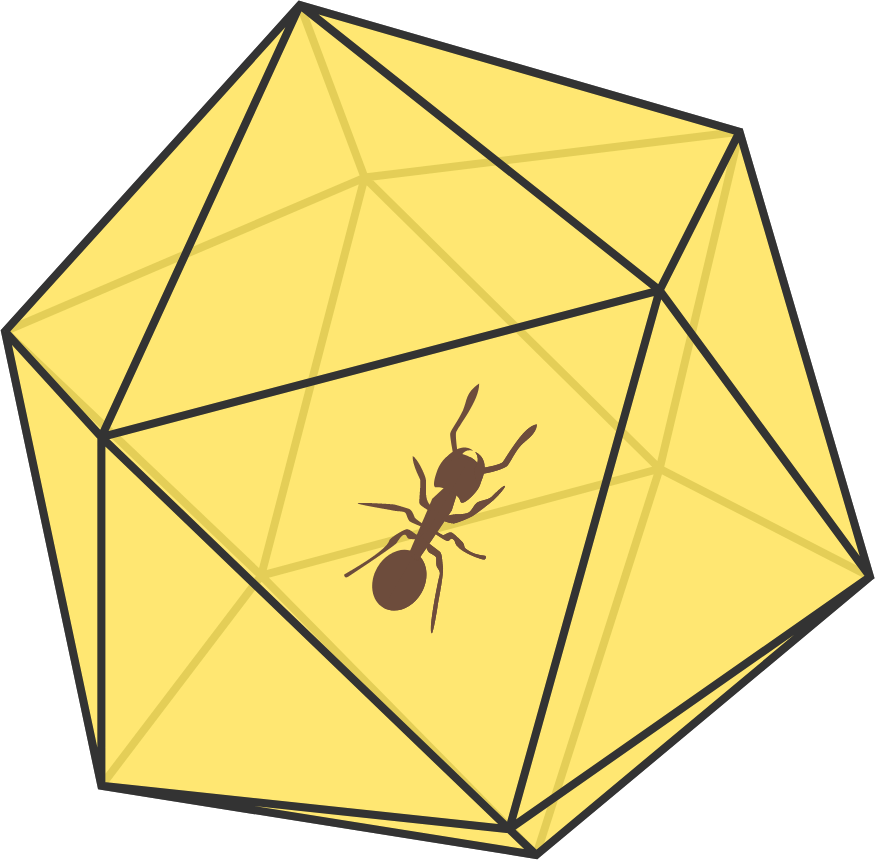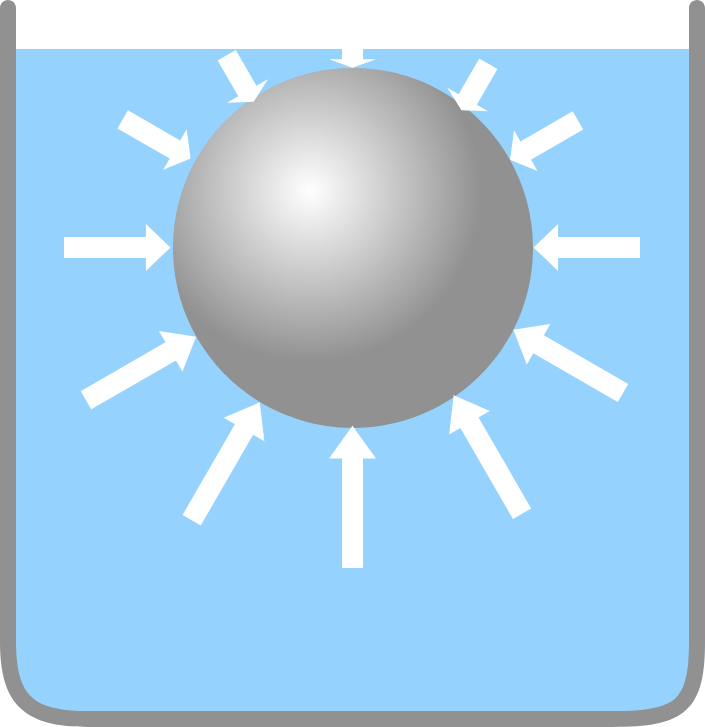# Problems of the Week

Contribute a problem

# 2017-11-13 Advanced

Let $G_n$ be the expected value of the geometric mean of $n$ real numbers independently and randomly chosen between 0 and 1.

What is $\displaystyle \lim_{n \rightarrow \infty} G_n ?$

Brilli the ant is standing on a face of a regular icosahedron. He wants to walk on every other face of the icosahedron exactly once, and then, in the next move, walk back to the face that he started from. How many ways are there for Brilli to do this?Details and Assumptions: Brilli starts on a fixed face on the icosahedron. Brilli will not walk along edges; he will only walk on an edge to cross to another face. You may find it useful to use the code environment below, which contains a dictionary that encodes the three faces adjacent to each face.

adjacencies = {
1 : [7, 13, 19], 2 : [12, 18, 20], 3 : [16, 17, 19], 4 : [11, 14, 18],
5 : [13, 15, 18], 6 : [9, 14, 16], 7 : [1, 15, 17], 8 : [10, 16, 20],
9 : [6, 11, 19], 10 : [8, 12, 17], 11 : [4, 9, 13], 12 : [2, 10, 15],
13 : [1, 5, 11], 14 : [4, 6, 20], 15 : [5, 7, 12], 16 : [3, 6, 8],
17 : [3, 7, 10], 18 : [2, 4, 5], 19 : [1, 3, 9], 20 : [2, 8, 14]
}
Python 3
You need to be connected to run codeThe sphere is just barely submerged in water.

Find the ratio of the hydrostatic force upon the bottom half of the sphere to the hydrostatic force upon the top half of the sphere.

Details and Assumptions:

• Assume the whole set up is in vacuum.

$f(x) = \begin{cases} 0 + \beta \ : \ \lfloor x \rfloor \equiv 0 \mod 3 \\ 1 + \beta \ : \ \lfloor x \rfloor \equiv 1 \mod 3 \\ 2 + \beta \ : \ \lfloor x \rfloor \equiv 2 \mod 3 \\ \end{cases}$

There is a unique value of $\beta$ such that $\displaystyle \sum_{n=1}^{\infty} \frac{f \left( 3^{n}\sqrt{2017} \right)}{3^{n}} = 0.$ This value of $\beta$ can be expressed as $a-b\sqrt{c},$ where $a,\ b,$ and $c$ are positive integers and $c$ is square-free. Find $a + b + c .$

$N$ particles with electric charge $q$ are placed on a unit sphere at random. They repel each other and relax toward an equilibrium configuration as their motion damps away.

In the code box below, design an algorithm that computes the potential energy of the equilibrium.


Details & Code:

• Enter your answer for $N=17$ in units where $\frac{q^2}{4\pi\epsilon_0}=1.$
• The function U(listofPoints) takes a list of NumPy arrays containing the position vectors of each of the $N$ charges, and returns the total potential energy $\sum\limits_{i=1}^N \sum\limits_{j \gt i}^N \frac{1}{r_{ij}}.$
  1 2 3 4 5 6 7 8 9 10 11 def U(listofPoints): N = len(listofPoints) #number of charges in list U = 0 #Loops through unique pairs of charges for i in range(N): for j in range(i + 1, N): r = listofPoints[i] - listofPoints[j] U = U + 1.0 / numpy.linalg.norm(r) return U 

import numpy


Python 3
You need to be connected to run code

×

Problem Loading...

Note Loading...

Set Loading...